Help protect the Great Barrier Reef with TensorFlow on Kaggle

Color Space Conversions

Overview

In computer vision, the selected color space could have a significant the performance of the model. While RGB is the most common color space, in manay situations the model performs better when switching to alternative color spaces such as YUV, YCbCr, XYZ (CIE), etc.

The tensorflow-io package provides a list of color space conversions APIs that can be used to prepare and augment the image data.

Setup

Install required Packages, and restart runtime

pip install -q tensorflow-io

The image example used in this tutorial is a cat in the snow, though it could be replaced by any JPEG images.

The following will download the image and save to local disk as sample.jpg:

ls -ls sample.jpg
% Total    % Received % Xferd  Average Speed   Time    Time     Time  Current
100 17858  100 17858    0     0   235k      0 --:--:-- --:--:-- --:--:--  235k
20 -rw-rw-r-- 1 kbuilder kokoro 17858 Oct 27 16:33 sample.jpg

Usage

Read and decode the image into a uint8 Tensor of shape (213, 320, 3)

import tensorflow as tf
import tensorflow_io as tfio

print(image.shape, image.dtype)
(213, 320, 3) <dtype: 'uint8'>

The image can be displayed by:

import matplotlib.pyplot as plt

plt.figure()
plt.imshow(image)
plt.axis('off')
plt.show()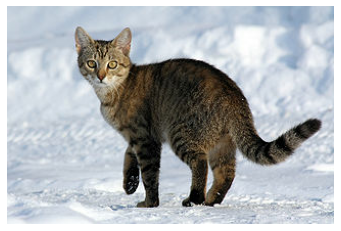Convert RGB to Grayscale

An RGB image can be converted to Grayscale to reduce the channel from 3 to 1 with tfio.experimental.color.rgb_to_grayscale:

grayscale = tfio.experimental.color.rgb_to_grayscale(image)

print(grayscale.shape, grayscale.dtype)

# use tf.squeeze to remove last channel for plt.imshow to display:
plt.figure()
plt.imshow(tf.squeeze(grayscale, axis=-1), cmap='gray')
plt.axis('off')
plt.show()
(213, 320, 1) <dtype: 'uint8'>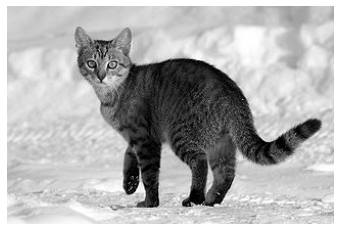Convert RGB to BGR

Some image software and camera manufacturors might prefer BGR, which can be obtained through tfio.experimental.color.rgb_to_bgr:

bgr = tfio.experimental.color.rgb_to_bgr(image)

print(bgr.shape, bgr.dtype)

plt.figure()
plt.imshow(bgr)
plt.axis('off')
plt.show()
(213, 320, 3) <dtype: 'uint8'>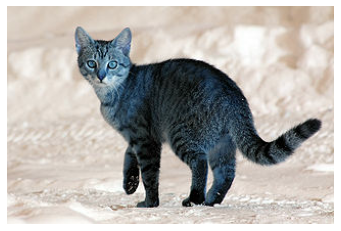Convert RGB to CIE XYZ

CIE XYZ (or CIE 1931 XYZ is a common color space used in many image processing programs. The following is the conversion from RGB to CIE XYZ through tfio.experimental.color.rgb_to_xyz. Note tfio.experimental.color.rgb_to_xyz assumes floating point input in the range of [0, 1] so additional pre-processing is needed:

# convert to float32
image_float32 = tf.cast(image, tf.float32) / 255.0

xyz_float32 = tfio.experimental.color.rgb_to_xyz(image_float32)

# convert back uint8
xyz = tf.cast(xyz_float32 * 255.0, tf.uint8)

print(xyz.shape, xyz.dtype)

plt.figure()
plt.imshow(xyz)
plt.axis('off')
plt.show()
(213, 320, 3) <dtype: 'uint8'>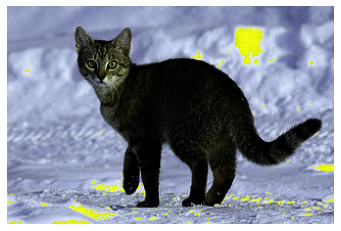Convert RGB to YCbCr

Finally, YCbCr is the default color space in many video systems. Converting to YCbCr could be done through tfio.experimental.color.rgb_to_ycbcr:

ycbcr = tfio.experimental.color.rgb_to_ycbcr(image)

print(ycbcr.shape, ycbcr.dtype)

plt.figure()
plt.imshow(ycbcr, cmap='gray')
plt.axis('off')
plt.show()
(213, 320, 3) <dtype: 'uint8'>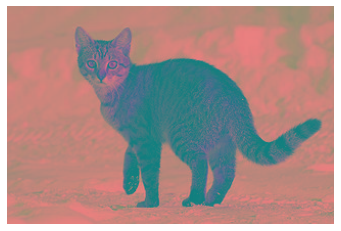What is more interesting, though, is that YCbCr could be decomposed into Y' (luma), Cb (blue-difference chroma), and Cr (red-difference chroma) components with each component carry perceptually meaningful information:

y, cb, cr = ycbcr[:,:,0], ycbcr[:,:,1], ycbcr[:,:,2]

# Y' component
plt.figure()
plt.imshow(y, cmap='gray')
plt.axis('off')
plt.show()

# Cb component
plt.figure()
plt.imshow(cb, cmap='gray')
plt.axis('off')
plt.show()

# Cr component
plt.figure()
plt.imshow(cr, cmap='gray')
plt.axis('off')
plt.show()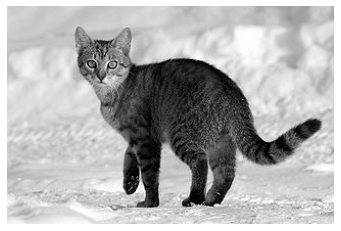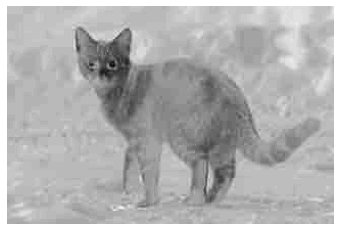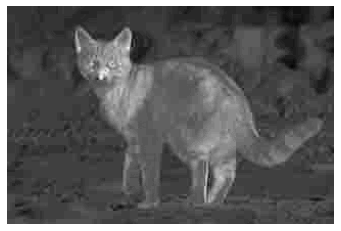[]
[]